# Column space#### Everything You Need in One Place

Homework problems? Exam preparation? Trying to grasp a concept or just brushing up the basics? Our extensive help & practice library have got you covered.#### Learn and Practice With Ease

Our proven video lessons ease you through problems quickly, and you get tonnes of friendly practice on questions that trip students up on tests and finals.#### Instant and Unlimited Help

Our personalized learning platform enables you to instantly find the exact walkthrough to your specific type of question. Activate unlimited help now!Get the most by viewing this topic in your current grade. Pick your course now.

##### Intros
###### Lessons
1. Column Space Overview:
2. definition of the column space
$C(A)=$ column space
• A set of vectors which span{$v_1,v_2,\cdots,v_n$}
$\vec{b} \;\epsilon \;C(A),$ $\vec{b}= x_1 v_1+x_2 v_2+\cdots+x_n v_n$
3. A vector in the column space
$\vec{b} =x_1 v_1+x_2 v_2+\cdots+x_n v_n$
• Changing to $Ax=b$ and solve
4. Finding a basis for the column space
• Row reduce the matrix to echelon form.
• Locate the columns with pivots in the row-reduced matrix.
• Go back to the original matrix and find the columns with the same position.
• Use those columns to write out the basis
##### Examples
###### Lessons
1. Finding if a vector is in the column space
Let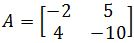and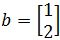. Determine whether $b$ is in the column space of $A$.
1. Let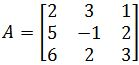and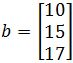. Determine whether $b$ is in the column space of $A$.
1. Finding a Basis for the Column Space
Here is the matrix $A$, and an echelon form of $A$. Find a basis for $C(A)$ (column space of $A$).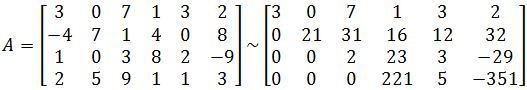1. Find a basis for the column space of $A$ if:###### Free to Join!
StudyPug is a learning help platform covering math and science from grade 4 all the way to second year university. Our video tutorials, unlimited practice problems, and step-by-step explanations provide you or your child with all the help you need to master concepts. On top of that, it's fun - with achievements, customizable avatars, and awards to keep you motivated.
• #### Easily See Your ProgressWe track the progress you've made on a topic so you know what you've done. From the course view you can easily see what topics have what and the progress you've made on them. Fill the rings to completely master that section or mouse over the icon to see more details.
• #### Make Use of Our Learning Aids###### Practice Accuracy

Get quick access to the topic you're currently learning.

See how well your practice sessions are going over time.

Stay on track with our daily recommendations.

• #### Earn Achievements as You LearnMake the most of your time as you use StudyPug to help you achieve your goals. Earn fun little badges the more you watch, practice, and use our service.
• #### Create and Customize Your AvatarPlay with our fun little avatar builder to create and customize your own avatar on StudyPug. Choose your face, eye colour, hair colour and style, and background. Unlock more options the more you use StudyPug.

### What is the column space of a matrix

Going back to the basic definition of a matrix, remember that we can think of a matrix as a collection of column vectors, this collection can be represented easily as:

Where $v_{1}, v_{2}, ..., v_{n}$ are the columns of A, each of them a column vector in itself.

This comes from the simple fact that our standard way to write matrices is in the form m x n, something we already know but is always good to remember, meaning that a matrix has m amount of rows and n amount of columns. This row and column standard gives us a little heads up to understand the notation above, since in order to know how many columns are there into our particular matrix we check for the highest value of n and that is where the range {$v_{1}, v_{2}, ..., v_{n}$} from equation 1 comes from.

The column space of a matrix A (also known as the range of a matrix) is then the set of vectors which contains all of the possible linear combinations of the vectors {$v_{1}, v_{2}, ..., v_{n}$}. Remember linear combinations mean you can have the column vectors multiplied by constant coefficients and the resulting vectors will be part of the column space of the matrix. Simply said, the column space of A can be defined as: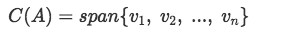Equation 2: Column space of matrix A

Where a span is the result of the multiplication of a scalar field with a vector field producing all the possible linear combinations of the vectors contained within the matrix in question. Before continuing, make sure you are certain of all the terms we are using so far, the term "linear combinations" happens to be a usual key point since it could be confused on its meaning depending on the importance given to either the value of a combination or the notation of its mathematical expression. In any way, linear combinations will be used in the simplest possible way during all our example exercises for the lesson but it is important that you have reviewed lessons such as linear independence and/or linear combination and vector equations before getting into the core for this topic.

Having looked at the column space definition above, we can identify if a certain vector is part of the column space of a matrix by checking if it is part of a possible linear combination resulting from A and its multiplication with a vector $\bar{x}$.

In other words, if vector $\bar{b}$ belongs to the column space of A (mathematically: if $\bar{b} \in C(A)$, then: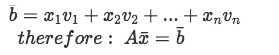Equation 3: Condition for a vector to be part of the column space of matrix A

Remember that C(A) is a substape of $R^{n}$, therefore, it fulfills the 3 properties of subspaces as well which we studied on the lesson named properties of subspace.

### How to find the column space of a matrix

Something nice to notice on this topic is that when learning how to find column space for a given matrix, you just need to look for all the possible linear combinations of the column vectors of the matrix. And so we just need to use the matrix equation represented in equation 3 to know that the matrix column space will contain all the range of possible results from the product of matrix A with a vector $\bar{x}$.

But the usual question happens to be if a certain vector belongs to the linear algebra column space of a known matrix. And so, in order to find out if a given vector is part of a column space we need to solve for the vector $\bar{x}$ in the matrix equation Ax=b (equation 3) and see if the result for such equation is consistent. Let us start by working a simple problem in order to come up with a set of steps to follow for our exercises below.

So, how do we check if vector $\bar{b}$ is in the column space of A?

Having: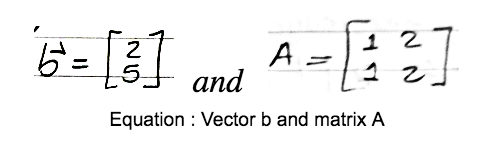Equation : Vector b and matrix A

We check for the condition expressed on equation 3 to see if vector $\bar{b}$ belongs to the column space of A: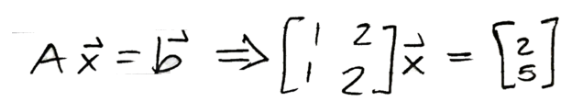Equation : Condition for b belonging to the column space of A

We turn this matrix equation into an augmented matrix to solve for vector $\bar{x}$:

Where we now row-reduce the augmented matrix to obtain the components of vector x: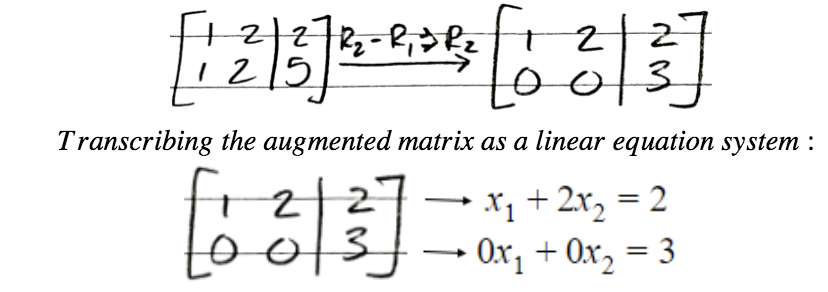Equation : Row-reducing the augmented matrix

Where you can easily see in the equation resulting from the second row that the whole system is inconsistent since zero plus zero cannot be equal to three!

And thus we can quickly determine that vector $\bar{b}$ does not belong to the column space of A.

After looking solving this simple example, let us write down the main steps to work through this process. And so, having a column vector b and a known matrix A:

1. We check if $\bar{b}$ belongs to the column space of A using the matrix equation Ax=b denoted in equation 3. If the result if consistent, then $\bar{b}$ belongs to the column space of A.
1. Set up the Matrix equation Ax=b with the given values for the matrix and the vector.
2. Turn the equation into an augmented matrix to solve for vector $\bar{x}$
3. Row-reduce the augmented matrix.
4. Obtain the components $x_{1}$ and $x_{2}$ of vector $\bar{x}$ by transcribing the linear equation system from the row reduction.
5. Check if the results are consistent.

We will be using these steps while solving example problems 1 and 2 in the example exercises section below.

### Find basis for column space

The second type of problem we will be solving throughout this lesson is that requiring you to find the basis for the column space of the given matrix.

The basis of column space in a matrix is the minimum set of vectors which are linearly independent in the span of the subspace which conforms the column space. Finding this basis is really simple and can be done following the next four steps:

1. Row reduce the matrix A to echelon form
2. Circle the columns with pivots in the row-reduced matrix
3. Go back to the original matrix and circle the columns with the same positions
4. Use these columns to write out the basis for C(A).

This process will be followed in order to find the basis for column space of the matrices given in problem examples 3 and 4 in the next section.

### Example Exercises

Example 1

Let A and $\bar{b}$ be as shown below. Determine whether $\bar{b}$ is in the column space of A.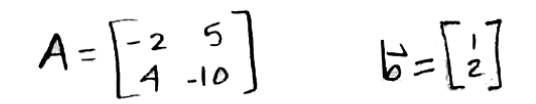Equation for example 1: Matrix A and vector b
• We start by setting up the condition shown in equation 3 and then we turn the matrix equation into an augmented matrix to solve for vector $\bar{x}$: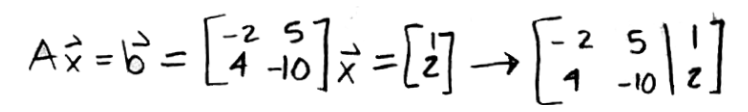Equation for example 1(a): Condition for b to belong to the column space of A
• Now we just row-reduce the augmented matrix to see if we find a consistent result.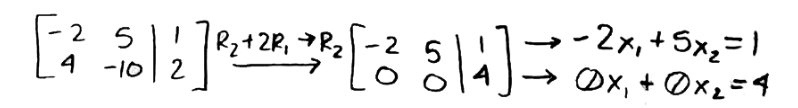Equation for example 1(b): Row reducing to find a set of linear equations

If we check the set of linear equations obtained from the row-reduction of the augmented matrix, we can clearly see how the values of the components are inconsistent since is impossible for 0+0 to be equal to 4! And so, since this result is incorrect, this means vector $\bar{b}$ does not belong to the column space of A.

Example 2

Now let us have A and $\bar{b}$ be as shown below. Determine if $\bar{b}$ is in the column space of A.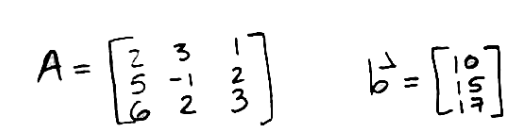Equation for example 2: Matrix A and vector b
• Once again, we set up the condition from the matrix equation Ax=b shown in equation 3 and then we turn it into an augmented matrix to solve for vector $\bar{x}$: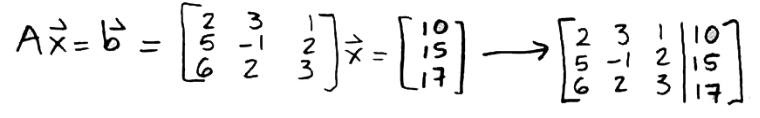Equation for example 2(a): Condition for b to belong to the column space of A
• Now we just row-reduce the augmented matrix to see if we find a consistent result.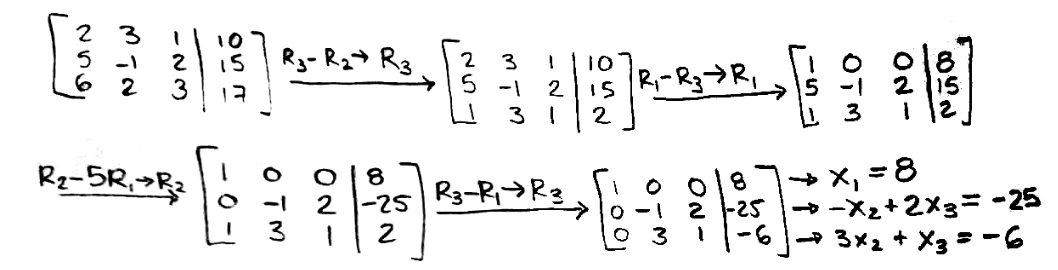Equation for example 2(b): Row reducing to find a set of linear equations
• In this case we have as a result a set of three linear equations, we now work through them and find the value for each of the components of the vector x. If the values are consistent, then this means b belongs to the column space of A, if the values do not make sense, then we would obtain the same as in example 1.
• Since the value for $x_{1}$ is already known, we just take the second and third equations from the set found above and solve for $x_{2}$ and $x_{3}$.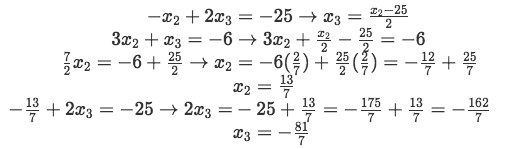Equation for example 2(c): Solving for the values of the components of x
• Having found the values of $x_{2}$ and $x_{3}$ we can now construct the whole vector $\bar{x}$.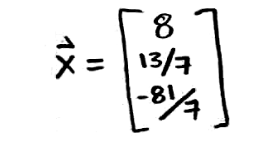Equation for example 2(d): Vector x
• The solution to the matrix equation is consistent. Therefore, vector $\bar{b}$ belongs to the column space of A.

Example 3

Find a basis for the column space C(A) when the matrix A is as follows: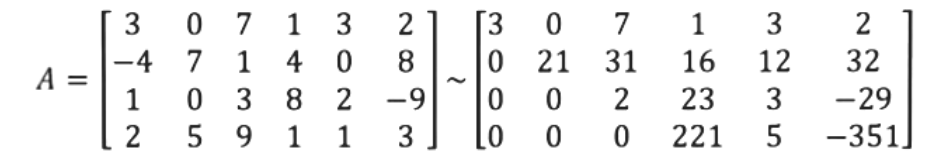Equation for example 3: Matrix A
• For this problem we use the steps described on the section "find basis for column space" above. Notice that for the case of this example problem, the echelon form of matrix A is already given to us (is the right hand side of the equation above), therefore, we do not have to work the row-reduction for this problem and we go directly into step 2: Circle the columns with pivots in the row-reduced matrix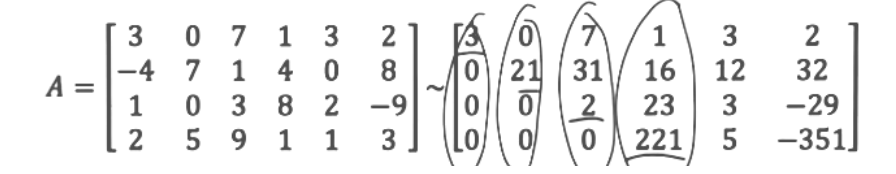Equation for example 3(a): Circling the pivots in the echelon form matrix
• The we go back to the original matrix and circle the columns with the same positions: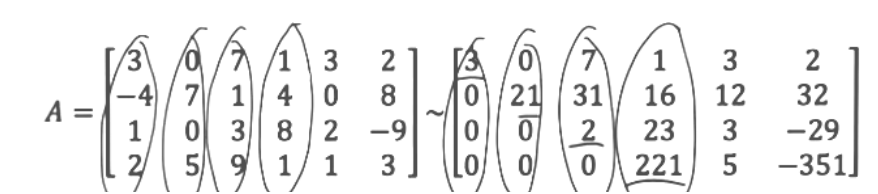Equation for example 3(b): Circling the proper positions in the original matrix A
• And finally, we use these columns to write out the basis for C(A).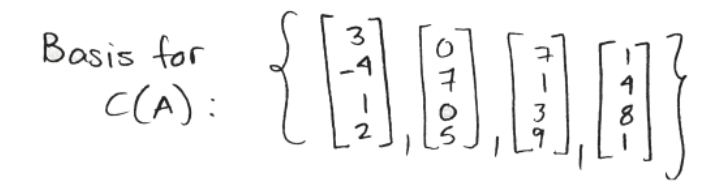Equation for example 3(c): Basis for C(A)

After looking at this example is important to note that it does not matter how many rows and columns matrix A has, the basis of a matrix column space can always be found since you can row-reduce the matrix into one of its echelon forms. If you want to practice and review row reduction and echelon forms do not hesitate to go back to that lesson and review its videos.

Example 4

Find the basis for column space of A if the matrix is as follows: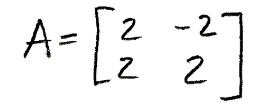Equation for example 4: Matrix A
• We start by transforming matrix A into its row echelon form by row reduction: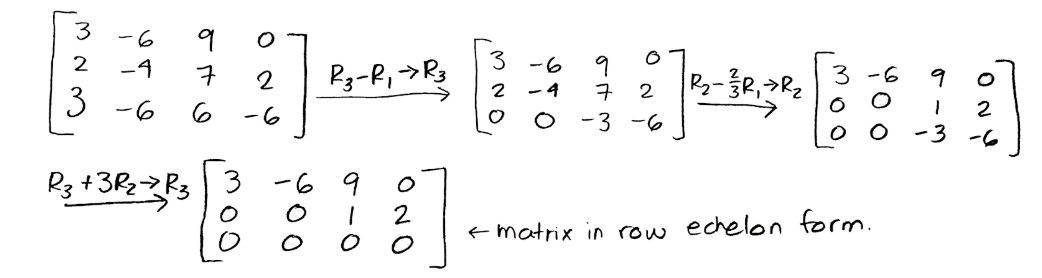Equation for example 4(a): Row reduction to produce the row echelon form matrix
• And so, we now have the original matrix and the row echelon form matrix: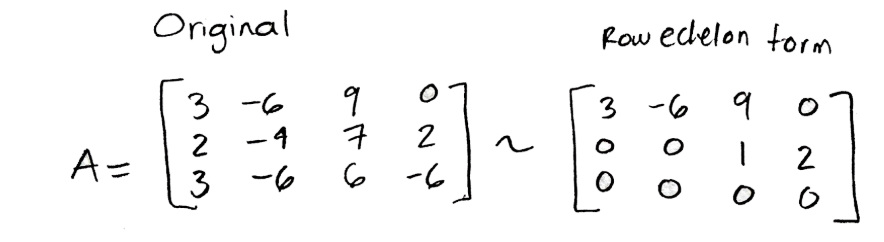Equation for example 4(b): Matrix A and its row echelon form
• Following step two we circle the columns with pivots in the row-reduced matrix: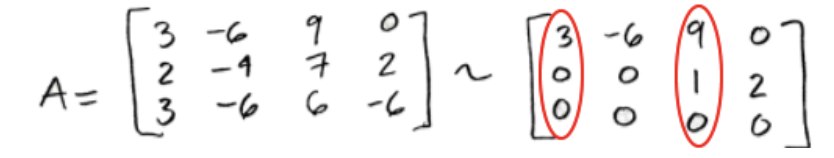Equation for example 4(c): Circling the pivots in the echelon form matrix
• Circling the same positions in the original matrix: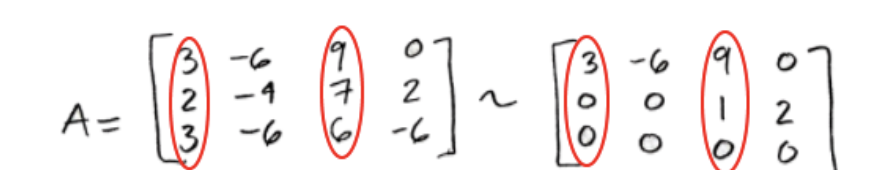Equation for example 4(d): Circling the proper positions in the original matrix A
• And so, we use these columns to write out the basis for C(A):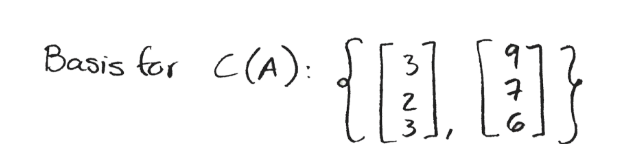Equation for example 4(e): Basis for C(A)

Having seen a few explicit examples, is time to go to the next lesson. If you would like to keep on your practice you can check out this article on row space and column space. Also, for an extra example that you can take a look while studying, we recommend you to look into this column space conditions problem.

The column space of a matrix $A$ is a subspace of $\Bbb{R}^n$.

Suppose the matrix $A$ is:

$A=[v_1\;v_2\; \cdots \;v_n ]$

where $v_1,v_2,\cdots,v_n$ are the columns of $A$. Then the column space of $A$ is the set of vectors in $C(A)$ which forms a linear combination of the columns of $A$.

To see if a vector $\vec{b}$ is in the column space of $A$, we need to see if $\vec{b}$ is a linear combination of the columns of $A$. In other words,
$\vec{b} =x_1 v_1+x_2 v_2+\cdots+x_n v_n$

where $x_1,x_2,\cdots,x_n$ are solutions to the linear equation.

To find a basis for the column space of a matrix A, we:
1) Row reduce the matrix to echelon form.
2) Circle the columns with pivots in the row-reduced matrix.
3) Go back to the original matrix and circle the columns with the same positions.
4) Use those columns to write out the basis for $C(A)$.

Note that the vectors in the basis are linearly independent.